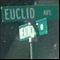Discussion about math, puzzles, games and fun.   Useful symbols: ÷ × ½ √ ∞ ≠ ≤ ≥ ≈ ⇒ ± ∈ Δ θ ∴ ∑ ∫  π  -¹ ² ³ °

You are not logged in.

## #1 2007-09-20 02:50:28

dannyv
MemberRegistered: 2007-09-20
Posts: 34

### Divide and Conquer Algorithms

Does anyone read the book "Algorithms" by Papadimitriou et al.? I found it to be an excellent book, just sometime need a little bit of explanation in some parts of it. For example, in chapter 2 about divide and conquer algorithms it presents a master theorem about the time complexity for this kind of algoritms. It saids something like this:

"If T(n)=aT(n/b)+O(n^d) for some constants a>0, b>1 and d>=0 then

T(n)= O(n^d)               if d>log_b(a)
T(n)= O(n^d log n)       if d=log_b(a)
T(n)= O(n^{log_b(a)}) if d<log_b(a)

Where n is the size of the input, a is the branching factor and b is how much we partition the input in each recursion step."

Can anyone tell me what is the parameter d?

Thanks

Offline

## #2 2007-09-20 04:23:22

Ricky
ModeratorRegistered: 2005-12-04
Posts: 3,791

### Re: Divide and Conquer Algorithms

When you have a divide and conquer algorithm, they do something on each step after they divide.  O(n^d) is the cost of what they do on this step.  So let's say my imaginary algorithm divides a list in half, then sorts each part of that list with bubble sort.  The cost of the bubble sort is n^2, so d = 2.

"In the real world, this would be a problem.  But in mathematics, we can just define a place where this problem doesn't exist.  So we'll go ahead and do that now..."

Offline

## #3 2007-09-20 04:58:37

dannyv
MemberRegistered: 2007-09-20
Posts: 34

### Re: Divide and Conquer Algorithms

Thanks, know is cristal clear!! :-)

Offline